# Semicircle

To a semicircle with diameter 10 cm inscribe square. What is the length of square sides?

s =  4.4721 cm

### Step-by-step explanation: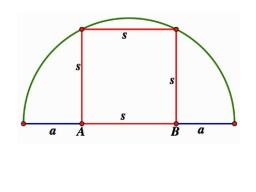Did you find an error or inaccuracy? Feel free to write us. Thank you!Tips to related online calculators
Looking for help with calculating roots of a quadratic equation?
Do you want to convert length units?
Pythagorean theorem is the base for the right triangle calculator.

#### You need to know the following knowledge to solve this word math problem:

We encourage you to watch this tutorial video on this math problem:

## Related math problems and questions:

• Rectangle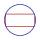The rectangle is 18 cm long and 10 cm wide. Determine the diameter of the circle circumscribed to the rectangle.
• Chord ABWhat is the chord AB's length if its distance from the center S of the circle k(S, 92 cm) is 10 cm?
• Concentric circles and chordIn a circle with a diameter d = 10 cm, a chord with a length of 6 cm is constructed. What radius have the concentric circle while touch this chord?
• Silver medal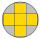To circular silver medal with a diameter of 10 cm is an inscribed gold cross, which consists of five equal squares. What is the content area of the silver part?
• LogsThe trunk diameter is 52 cm. Is it possible to inscribe a square prism with side 36 cm?
• Ratio of sidesCalculate the area of a circle with the same circumference as the circumference of the rectangle inscribed with a circle with a radius of r 9 cm so that its sides are in ratio 2 to 7.
• Circle chordCalculate the length of the chord of the circle with radius r = 10 cm, length of which is equal to the distance from the center of the circle.
• Chord distanceThe circle k (S, 6 cm), calculate the chord distance from the center circle S when the length of the chord is t = 10 cm.
• Find the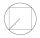Find the length of the side of the square ABCD, which is described by a circle k with a radius of 10 cm.
• Circumscribed circle to squareFind the length of a circle circumscribing a square of side 10 cm. Compare it to the perimeter of this square.
• Tree trunk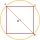What is the smallest diameter of a tree trunk that we can cut a square-section square with a side length of 20 cm?
• Diameter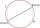If the endpoints of a diameter of a circle are A(10, -1) and B (3, 10), what is the radius of the circle?
• Rhombus and inscribed circleIt is given a rhombus with side a = 6 cm and the radius of the inscribed circle r = 2 cm. Calculate the length of its two diagonals.
• TangentsTo circle with a radius of 41 cm from the point R guided two tangents. The distance of both points of contact is 16 cm. Calculate the distance from point R and circle centre.
• Square circles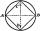Calculate the length of the described and inscribed circle to the square ABCD with a side of 5cm.
• Logs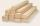The log has a diameter 30 cm. What's largest beam with a rectangular cross-section can carve from it?
• Square and circle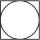Into square is an inscribed circle with diameter 10 cm. What is the difference between circumference square and circle?